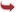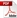International Journal of Scientific and Research Publications

#### IJSRP, Volume 6, Issue 3, March 2016 Edition [ISSN 2250-3153]A Comparison of Chebyshev polynomials and Legendre polynomials in order to solving Fredholm integral equations
Iman Malmir
Abstract: In this research we use the numerical solution method that is based on Chebyshev polynomials and Legendre polynomials, to solve non-singular integral equation, it is known as Fredholm integral equation of the second kind. We use these expansions because of their convergence and recurrence properties. Also both of them can be represented as trigonometric function on [1, -1]. First, we expand the unknown function in the integral equation based on the related formulas, then develop kernel of integral equation. To find these, we should try to find a function which can be represented as the solution of linear differential equations. Then substitution into the integral equation, we find the coefficients of the function. At the end of research the method will be illustrated by the mean of an example.
[VIEW FULL PAPER][DOWNLOAD]

Reference this Research Paper (copy & paste below code):

Iman Malmir (2018); A Comparison of Chebyshev polynomials and Legendre polynomials in order to solving Fredholm integral equations; Int J Sci Res Publ 6(3) (ISSN: 2250-3153). http://www.ijsrp.org/research-paper-0316.php?rp=P515139